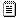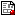[ Data Screening || Matrix Algebra with SAS/IML || Regression analysis || General Linear Models || Canonical correlation, Discriminant analysis || Logistic Regression || Factor Analysis || Clustering and scaling || SAS macro programs || Data sets || Programs]

## Data Screening

automiss.sasDemonstrates some ways of handling missing data, not all good.
basesca1.sasScatterplot matrix of baseball data. Illustrates the use of the SCATMAT macro for scatterplot matrices.
outldemo.sasMultivariate outlier detection Mammals Teeth data. Illustrates use of the OUTLIER macro to detect multivariate outliers.
outldem1.sasOutlier demonstration: 2 & 3 variables. Illustrates the problem of multivariate outliers in simple 2- and 3-variable situations, where univariate or even bivariate plots may not show anything unusual.
outldem2.sasOutlier detection: Simple ChiSquare Probability plot. A continuation of OUTLDEM1, this example shows the basic idea behind the outlier plot.
outltest.sas?? Multivariate outlier detection: Mammals Teeth data
poolscp.sasWithin and Between Group SSCP. Generates some random data to show how group differences in means can affect the Variance-Covariance matrix.
poolscpg.sasA continuation of POOLSCP, with a Proc GPLOT display, showing the effects of group differences on Variance Covariance matrix
scatautp.sasScatterplot matrices with PROC PLOT. They're not the best, but they are fairly easy to do for a quick look at a multivariate data set.